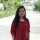Java Developers
Group for Java developers and programmers. Ask questions and get answers related to Java programming
253 Members
Join this group to post and comment.Computer Science
08 Feb 2019

# Bitwise operators in java

Bitwise operators are used to perform manipulation of individual bits of a number. They can be used with any of the integral types.

|    Bitwise OR -   if either of the bits is 1, it gives 1, else it gives 0.

&    Bitwise AND-  if both bits are 1, it gives 1, else it gives 0.

~    Bitwise Complement -all bits inversed, means it makes every 0 to 1, and every 1 to 0.

^    Bitwise XOR - if corresponding bits are different, it gives 1, else it gives 0.

<<    Left Shift -Shifts the bits of the number to the left and fills 0 on voids left as a result.

>>    Right Shift -Shifts the bits of the number to the right and fills 0 on voids left as a result.

>>>    Unsigned Right Shift -Shifts the bits of the number to the right and fills 0 on voids left as a result. The leftmost bit is set to 0. (>>>) is unsigned-shift; it’ll insert 0. (>>) is signed, and will extend the sign bit.

Suppose :a = 60, b = 13

a & b = 12

a | b = 61

a ^ b = 49

~a = -61

a << 2 = 240

a >> 2  = 15

a >>> 2 = 15